International
Tables for
Crystallography
Volume B
Reciprocal space
Edited by U. Shmueli

International Tables for Crystallography (2006). Vol. B, ch. 5.1, pp. 535-536   | 1 | 2 |

## Section 5.1.2.2. Wavefields

A. Authiera*

aLaboratoire de Minéralogie-Cristallographie, Université P. et M. Curie, 4 Place Jussieu, F-75252 Paris CEDEX 05, France
Correspondence e-mail: authier@lmcp.jussieu.fr

#### 5.1.2.2. Wavefields

| top | pdf |

The notion of a wavefield, introduced by Ewald (1917), is one of the most fundamental concepts in dynamical theory. It results from the fact that since the propagation equation (5.1.2.2)is a second-order partial differential equation with a periodic interaction coefficient, its solution has the same periodicity,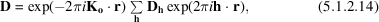where the summation is over all reciprocal-lattice vectors h. Equation (5.1.2.14)can also be written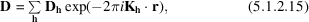where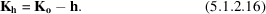Expression (5.1.2.15)shows that the solution of the propagation equation can be interpreted as an infinite sum of plane waves with amplitudes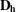and wavevectors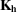. This sum is a wavefield, or Ewald wave. The same expression is used to describe the propagation of any wave in a periodic medium, such as phonons or electrons in a solid. Expression (5.1.2.14)was later called a Bloch wave by solid-state physicists.

The wavevectors in a wavefield are deduced from one another by translations of the reciprocal lattice [expression (5.1.2.16)]. They can be represented geometrically as shown in Fig. 5.1.2.1(b). The wavevectors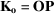;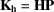are drawn away from reciprocal-lattice points. Their common extremity, P, called the tie point by Ewald, characterizes the wavefield.

In an absorbing crystal, wavevectors have an imaginary part,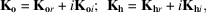and (5.1.2.16)shows that all wavevectors have the same imaginary part,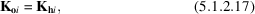and therefore undergo the same absorption. This is one of the most important properties of wavefields.

### References

Ewald, P. P. (1917). Zur Begründung der Kristalloptik. III. Röntgenstrahlen. Ann. Phys. (Leipzig), 54, 519–597.Google Scholar